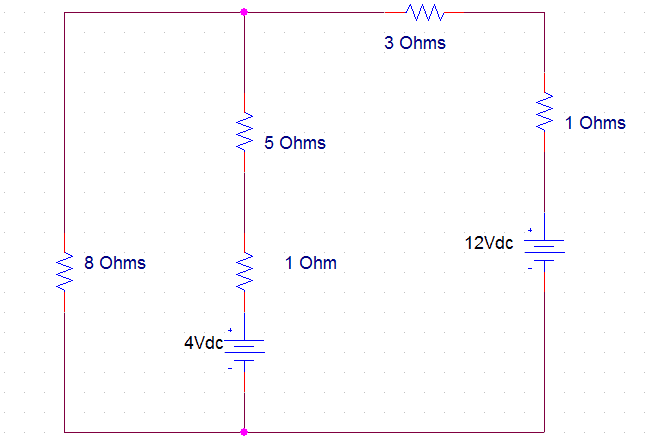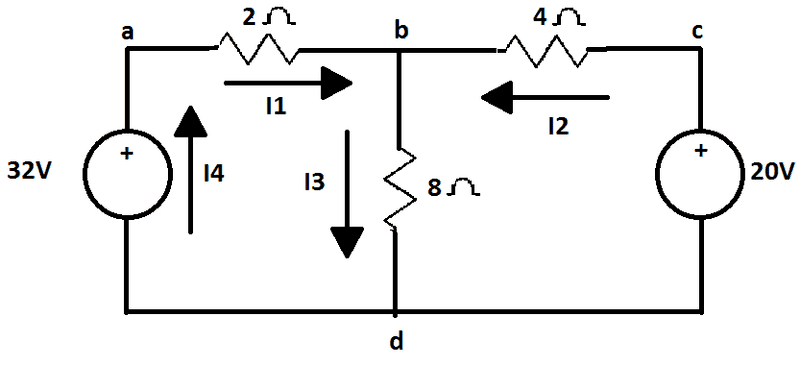# Simple Circuit Problem

• Engineering

## Homework Statement

What is the current through the 3 Ohms resistor in the diagram below?## Homework Equations

I=V/R and Kirchoff's Rule

## The Attempt at a Solution

I tried to simplify the circuit by adding the 5ohm and 1ohm resistors because they are in series, an the same thing with the 3ohm and 1ohm resistor. This problem has two voltage source so it's kind of confusing. I think I may have to use Kirchoff's Rules but I'm not sure how to approach this to get the answer. Any help would be great.

So the best way to answer this without just giving you the answer it to solve a similar question so with that in mind, I will use this circuit.There are other methods out there but I'm going to use the Branch-Current method (look up loop current method).

Step 1 is to define the branch currents and the direction that they are acting in.
Steb 2 Define the voltages in terms of the currents
$$V_{ab} = 2I_1$$
$$V_{bd} = 8I_3$$
$$V_{cb} = 4I_2$$

Step 3 using Kirchoffs Voltage law we get

$$ƩV_{abda} = 0 = V_{ab} + V_{bd} + V_{da}$$
$$ƩV_{bcdb} = 0 = V_{cb} + V_{bd} + V_{dc}$$

These can be re written as

$$ƩV_{abda} = V_{ab} + V_{bd} + V_{da} = 2I_1 + 8I_3 - 32$$
$$ƩV_{bcdb} = V_{cb} + V_{bd} + V_{dc} = 4I_2 + 8I_3 - 20$$

then finally You use Kichchoffs current laws at node b to get your final equation.

$$ƩI_b = 0 = I_1 + I_2 - I_3$$

this gives the 3 simultaneous equations

$$I_1 + I_2 - I_3= 0$$
$$2I_1 + 8I_3 = 32$$
$$4I_2 + 8I_3 = 20$$

Solving gives the currents $I_1 = 4$, $I_2 = -1$ and $I_3= 3$.

Hope that helps you.

rude man
Homework Helper
Gold Member
1This problem has two voltage source so it's kind of confusing.

If two voltage sources confuse you, this is an excellent time to invoke the Superposition theorem.

So make the first source zero volts (short) and calculate the current thru the 3 ohm = i1.

Then repeat, shorthing out the second source & working with the first. Calculate i2.

Then when both sources are active the answer is i1 + i2.